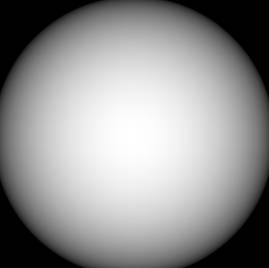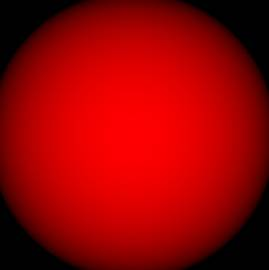### Adding color map type inputs and outputs to a GPU node

You can create new custom node inputs and outputs that support color maps.

Right-click in an open custom node graph and select Edit > Create node > New custom node > Inputs or Outputs and choose color map.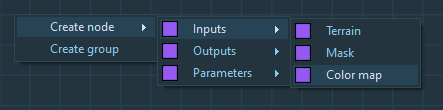Example: This node has a color map input named Input and a color map output named Output.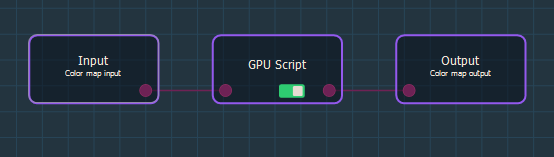### Handling colors

In the script, to get the color of the current point of a color map, use the following syntax to declare a Color type variable:

```// Declaration of a Color type variable and getting the color of the current point
Color C = Input
```

From a Color type variable, it is possible to retrieve the R, G, and B channels using the Red, Green, and Blue functions:

```// Store the channels into 3 different variables
r = Red(c)
g = Green(c)
b = Blue(c)
```

The r, g, b variables can now be updated.

To store the result in the output color map, use the Color function. It uses three parameters and returns a Color value.

```//Store into the output color map
Output = Color(r,g,b)
```

#### Example of script converting a color map into gray levels

```Color col = Input

r = Red(col)
g = Green(col)
b = Blue(col)

brightness = 0.3 * r + 0.59 * g + 0.11 * b

coef = Intensity / 100
rFinal = (1 - coef) * r + coef * brightness
gFinal = (1 - coef) * g + coef * brightness
bFinal = (1 - coef) * b + coef * brightness

Output = Color(rFinal, gFinal, bFinal)
```

Note: Do not name a variable "color" as this will conflit with the Color function.

Note: When the "Can access other points" option is used, the value of an input color map's point will be retrieved with syntax Input[dx,dy] instead of Input, which is the same as for terrain or mask inputs.

#### Updating a terrain color map

When using a terrain input and output, it is possible to update the terrain color map when present.
To get the input or output terrain's color map, just suffix its name with _ColorMap.

For example, if the input terrain's name is Input, its color map is named Input_ColorMap.
If the output terrain's name is Output, its color map is named Output_ColorMap.

Example of script modifying a terrain and its color map:

```// Add 100 meters to the elevation
Output = Input + 100

// Only keep the red channel
Color c = Input_ColorMap
Output_ColorMap = Color(Red(c), 0, 0)
```

If the input terrain has no color map, the instructions about the color maps are ignored at runtime.

#### Mixing different input and output types

It is possible to define different types for the input and the output.

This node has a color map input and a Mask output.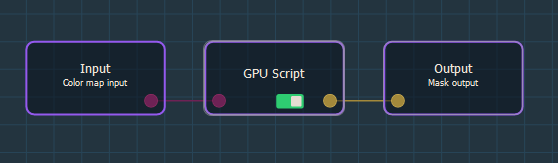With the following script, you create a mask from the input color map's red channel.

```Output = Red(Input)
```

In this example, the node has a Mask input and a color map output.With the following script, you create a color map from the red channel, which contains the mask value.

```Output = Color(Input,0,0)
```